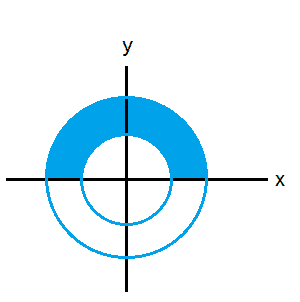# Double Integral in polar coordinates

says

## Homework Statement

Evaluate ∫∫D (3x + 4y 2 ) dA, where D = {(x, y) : y ≥ 0, 1 ≤ x 2 + y 2 ≤ 4} with the use of polar coordinates.

## The Attempt at a Solution

I made a sketch of the circle. It's radius is = 1 and it's lowest point is at (0,0), highest at (0,2), leftmost point at (-1,1) and rightmost point at (1,1).

Converting this integral into polar coordinates:
∫∫D (3rcosθ+4(rsinθ)2) r dr dθ

then distributing the r

∫∫D (3r2cosθ+4r(rsinθ)2) dr dθ

The outside integral goes from 0≤θ≤2π
I'm not totally sure with the inside integral though.
0≤r≤1? Because the maximum that the radius is is 1?

I haven't evaluated it yet. I want to make sure I've set it up correctly first. :)

Homework Helper
Gold Member

## Homework Statement

Evaluate ∫∫D (3x + 4y 2 ) dA, where D = {(x, y) : y ≥ 0, 1 ≤ x 2 + y 2 ≤ 4} with the use of polar coordinates.

## The Attempt at a Solution

I made a sketch of the circle. It's radius is = 1 and it's lowest point is at (0,0), highest at (0,2), leftmost point at (-1,1) and rightmost point at (1,1).

Converting this integral into polar coordinates:
∫∫D (3rcosθ+4(rsinθ)2) r dr dθ

then distributing the r

∫∫D (3r2cosθ+4r(rsinθ)2) dr dθ

The outside integral goes from 0≤θ≤2π
I'm not totally sure with the inside integral though.
0≤r≤1? Because the maximum that the radius is is 1?

I haven't evaluated it yet. I want to make sure I've set it up correctly first. :)

Have you drawn a picture of the full region yet? Isn't there more than one circle? What does the region ##D## look like?

says
Sorry, I just realised there are a few formatting errors in my original post.

Evaluate ∫∫D (3x + 4y2 ) dA, where D = {(x, y) : y ≥ 0, 1 ≤ x2 + y2 ≤ 4} with the use of polar coordinates.

I only got one circle. But I've seen that I've made a mistake. I'm having a lot of trouble interpreting what D means and plotting the graph. :/

I take D to mean that y is always greater than or equal to 0, so the region isn't below 0, and that x2 + y2 is between 1 and 4. But with this interpretation I get a 4 triangles, which doesn't seem right...

Homework Helper
Can you sketch x2+y2=1? what curve is it? And what is x2+y2=4?

says
Can you sketch x2+y2=1? what curve is it? And what is x2+y2=4?

I can sketch both, I don't understand what you mean by 'what curve is it?' It's a circle, but D states that y has to be equal to or greater than 0, and that x2+y2 is between 1 and 4.

Homework Helper
I can sketch both, I don't understand what you mean by 'what curve is it?' It's a circle, but D states that y has to be equal to or greater than 0, and that x2+y2 is between 1 and 4.
Both are circles. What are the centres and the radii?

says
The radius of x2+y2=1 is 1, and for the other the radius = 2.

So the upper limit is 2 and the lower limit is 1. I should get two circles with this D?

says
Ok, I understand. d is saying:

x2 + y2 =1
x2 + y2 =4

Then the upper limit of the radius = 2 and lower limit = 1

Homework Helper
The radius of x2+y2=1 is 1, and for the other the radius = 2.

So the upper limit is 2 and the lower limit is 1. I should get two circles with this D?
The limits for what?
D is two-dimensional, you have an other variable, the angle θ. What are the limits for it?
Have yo sketched those circles?

says
The limit of integration.
For the angle θ, because y ≥ 0, the limit of integration is between 0≤θ≤π

Homework Helper
View attachment 99614
The limit of integration.
For the angle θ, because y ≥ 0, the limit of integration is between 0≤θ≤π
Correct. So the blue region is D.
Convert x, y into polar coordinates.says
3x + 4y2
∫∫(3rcosθ+4(rsinθ)2) r dr dθ
distribute the r
∫∫(3r2cosθ+4r(rsinθ)2) dr dθ

Homework Helper
Correct, go ahead. You want to integrate with respect to r first, what do you get?